# Long Division and Remainders

Long division is the process of writing out the division of one number by another. You will need to be able to perform long division on the GMAT. As often as possible, we’ll avoid computation on GMAT problems by estimating and looking for shortcuts. But on some problems you may have to divide one number by another without the use of a calculator. (Again, the Integrated Reasoning section has a built-in calculator, but the Quantitative section, which is more important, does not.)

1. Write the division so that the numerator goes “into” the denominator. In long division, we write the following division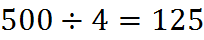as follows: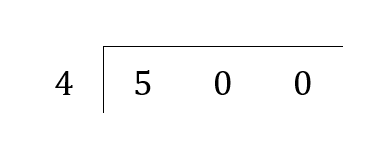In this example, 500 is the dividend and 4 is the divisor. If you get confused about which number goes in which place, recall that we want to see how many times 4 “goes into” 500. That means that 500 is inside the box and 4 is on the outside, “going in.”

2.  Count how many times the outer number goes into the first digit. Consider the leftmost digit of the dividend. Here, the dividend is 500, so the leftmost digit is 5. Find the largest multiple of the divisor that is less than the leftmost digit: in other words, mentally perform “5 divided by 4.” If the divisor doesn’t go into the leftmost digit, you must divide it into the first two digits of the dividend.

In this case, the largest multiple of 4 that is less than 5 is 4. In other words, 4 goes into 5 a maximum of 1 time. So we write 1 above the 5, for “1 time.”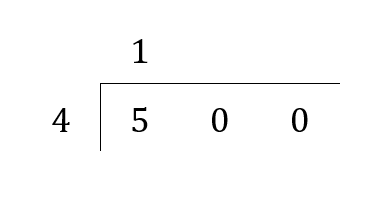3. Multiply the outer numbers and write the product under the first digit or digits. We multiply 1 by 4 to get 4, which we write under the 5.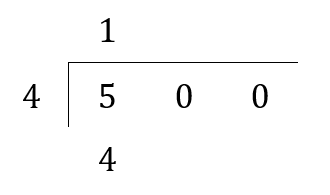Note that, when we multiply to get the number that goes under 5 here, we cannot obtain a number that is bigger than 5. If we tried putting a 2 up top, we would know we made a mistake when we multiplied 2 times 4 and tried putting 8 under the 5, because we aren’t allowed to put something larger than 5 under the 5.

4. Subtract and bring down a digit. Subtract the digit under the dividend from the digit used in the dividend–in this case, subtract 4 from 5. Write the result of that subtraction–which is 1, here–underneath, and bring down the next digit of the dividend–in this case, the first zero of 500. This gives you a new number to divide into–in this case, 10.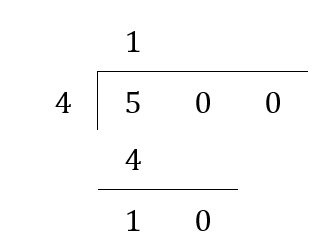5. Count how many times the divisor (the outer number) goes into the new number at the bottom. Now apply the same steps 2 and 3 to this new number, and write the results in the corresponding columns (in this case, the unit column is aligned with the second digit of the original dividend): multiple and remainder underneath the new number, and the answer above the line.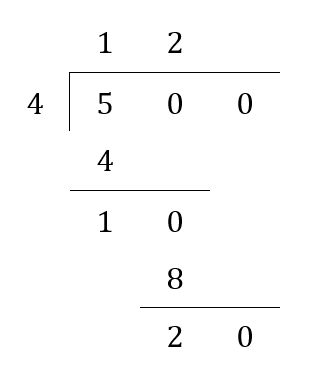6. Repeat until you have a remainder of zero, or sufficient accuracy. Repeat until there are no digits remaining in the dividend. The number written above the bar is the quotient, and the last remainder calculated is the remainder for the entire problem. Here, we end with a zero at the bottom, so the remainder is zero.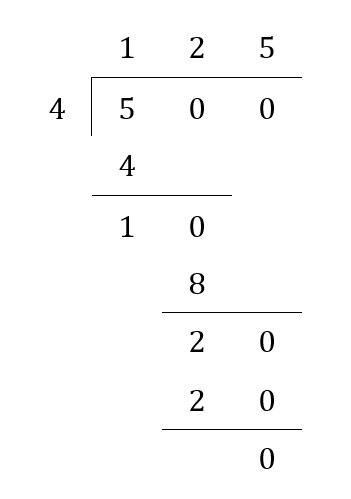## Exercise

Try dividing 3 by 7 using long division.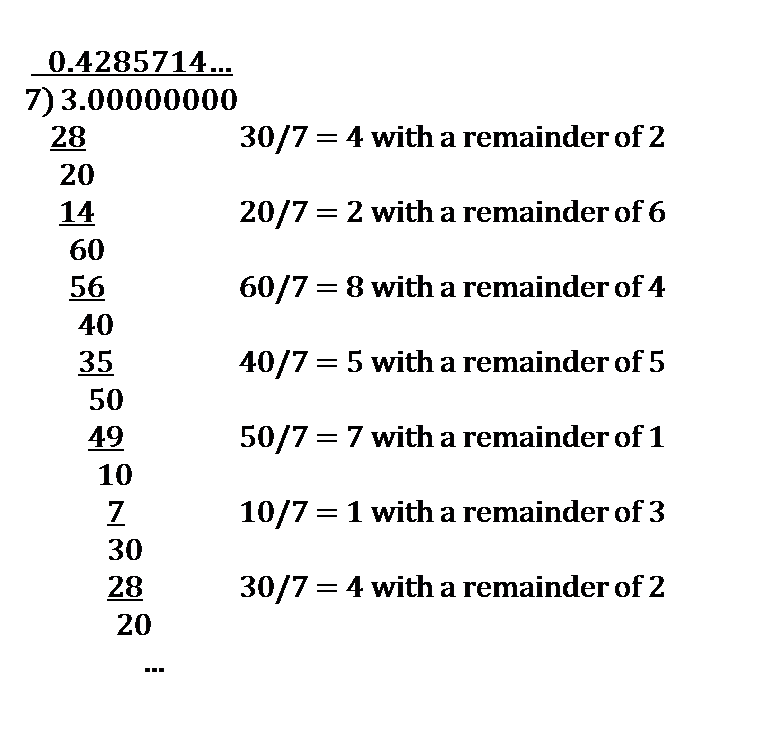You can try this over and over, confirming your work with a calculator, until you can perform long-division with total comfort.

## Practice Questions

Remainder and Fractional Portion:
http://www.gmatfree.com/Remainder-and-Fractional-Portion

Counting Zeroes:
http://www.gmatfree.com/Counting-Zeroes

Counting Nonzero Digits:
http://www.gmatfree.com/Counting-Nonzero-Digits

Welcome! You are encouraged to register with the site and login (for free). When you register, you support the site and your question history is saved.